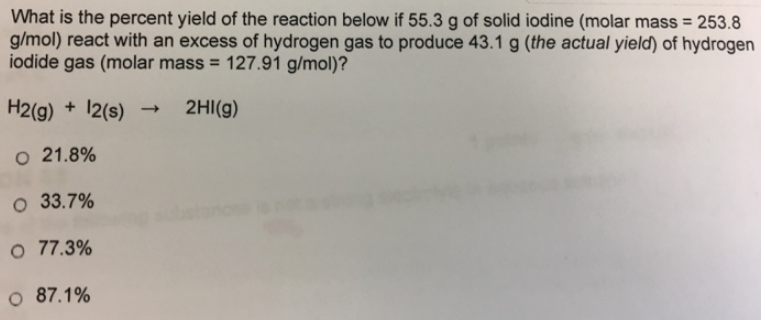# Problem: What is the percent yield of the reaction below if 55.3 g of solid iodine (molar mass = 253.8 g/mol) react with an excess of hydrogen gas to produce 43.1 g (the actual yield) of hydrogen iodide gas (molar mass = 127.91 g/mol)? H2 (g) + I2 (s) → 2HI (g) (i) 21.8% (ii) 33.7% (iii) 77.3% (iv) 87.1%

###### FREE Expert Solution
95% (203 ratings)###### Problem Details

What is the percent yield of the reaction below if 55.3 g of solid iodine (molar mass = 253.8 g/mol) react with an excess of hydrogen gas to produce 43.1 g (the actual yield) of hydrogen iodide gas (molar mass = 127.91 g/mol)?

H2 (g) + I(s) → 2HI (g)

(i) 21.8%

(ii) 33.7%

(iii) 77.3%

(iv) 87.1%